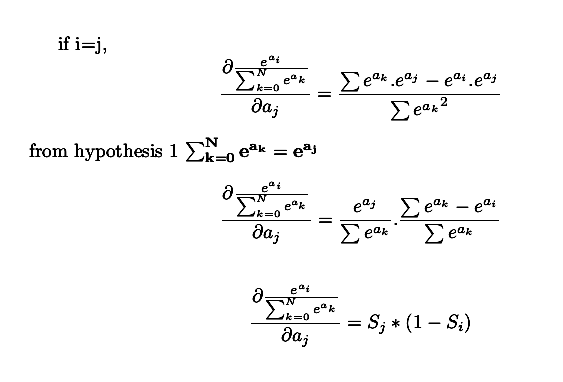# demystifying derivatives of softmax -2

Source: Deep Learning on Medium# demystifying derivatives of softmax -2

stage-2 of peeking into derivatives of softmax

HELLO FRIEND !! you are now at stage 2 of computing derivatives of softmax function. It is recommended to check stage1 before starting here.

!! Disclaimer !! this is my first technical article and open to constructive criticism.

continuing from a couple of hypothesis we framed in stage1 , we have to apply these hypothesis to compute the jacobians under condition i==j and i!=j.

I understand friend , you could be bored with equations. Now it’s time to prove the above properties. Torch is coming !!!!

`### empty array to store jacobians ###jb=np.empty(shape=(a.shape,a.shape))### the trivial part is here. we cannot compute jacobians w.r.t ### multiple inputs . hence we loop grads w.r.t each input and store ### it in above empty array.for i in range(a.shape): jb[i]=torch.autograd.grad(outputs = s[i], inputs = a,grad_outputs=torch.ones_like(a),retain_graph=True)print(jb)### array([[ 0.12033208, -0.0799501 , -0.04038198], ### [-0.0799501 , 0.24489387, -0.16494377], ### [-0.04038198, -0.16494377, 0.20532575]])`

We can verify above properties by substituting the values of (Si,Sj) and comparing them with jacobian matrices. See you in another post. GoodBye Friend !!!.